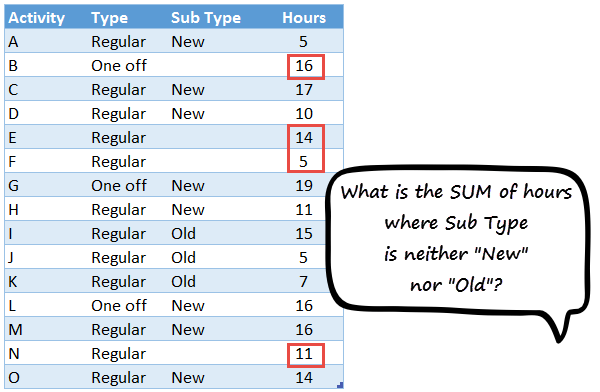# Sum up neither “A” nor “B” values – How to use DSUM function in Excel [video]

Share

We know how to use SUMIFS function to answer questions like, “What is the sum of values for ‘A’?”  But how would you answer questions like,

• What is the sum of values that are neither “A” nor “B”?

We can still use SUMIFS, but it will get awfully long. So let’s turn our attention to other functions in Excel.You can use either DSUM or SUMPRODUCT to answer questions like this.

### Using DSUM function to sum up neither “A” nor “B” values

Check out below video to understand how to use both DSUM & SUMPRODUCT formulas to calculate sum of neither “A” nor “B” values.

You can also watch this video on our YouTube Channel.

### More formula examples:

Check out below formula examples to up your Excel game.

### Don’t be a beginner OR newbie, master Excel with this awesome program

Excel skills can help you immensely at work and make you popular. So why struggle as a beginner. Become awesome in Excel today with our Excel School program. This comprehensive online course will teach you,

• Data analysis with Excel formulas
• Visual analysis with Charts
• Interactive analysis with Pivot tables, form controls
• Management / Exec reporting with Excel dashboards
• Productivity with key board shortcuts & ninja tricks

Check out Excel School program page for full details and enrollment options.

### Get FREE Excel + Power BI Tips

Simple, fun and useful emails, once per week.

Learn & be awesome.

### Welcome to Chandoo.org

Thank you so much for visiting. My aim is to make you awesome in Excel & Power BI. I do this by sharing videos, tips, examples and downloads on this website. There are more than 1,000 pages with all things Excel, Power BI, Dashboards & VBA here. Go ahead and spend few minutes to be AWESOME.

Read my storyFREE Excel tips book

I will be presenting at two events in London in April 2020. Come & join me.

Chandoo is an awesome teacher
5/5

– Jason

Still on fence about Power BI? In this getting started guide, learn what is Power BI, how to get it and how to create your first report from scratch.

### 10 Responses to “Sum up neither “A” nor “B” values – How to use DSUM function in Excel [video]”

1.NARAYAN says:

Hi ,

The SUMIFS usage seems more intuitive than the SUMPRODUCT one , at least in this case.

=SUMIFS(data[Hours],data[Sub Type],L6,data[Sub Type],M6)

=SUMIFS(data[Hours],data[Type],K6,data[Sub Type],L6,data[Sub Type],M6)

make use of the criteria as specified.

•Chandoo says:

Cool. I didn't know we can use same column twice in SUMIFS like this. Surely, this is a better way to solve the problem. Thanks for sharing.

•A Rajan Pradeep says:

Hi, has Pivots and Tables replaced quite a few "old world" formulas?

2.A Rajan Pradeep says:

Dear Chandoo,
When you have tables as you have here, cant this be better and done faster with just one formula - subtotal 109. You just have to select your preferences from the drop down menu.

3.Stef@n says:

Hi, another solution is: using Pivot :)))))) Regards Stef@n

4.Ghanshyam says:

Hi,
Thanks Chandoo. Very useful formula. But i have one question that i want to sum which is blank in sub type by DSUM function. How can i do it?

•Nathan says:

Blanks (empty cells) in Excel are referenced using double quotes - ""

I'm not terribly familiar with DSUM, but most likely the value you would want in the box is:

=""

Since the syntax looks like [Logical Operator][Logical Test]

Note that this should only catch things that are actually blank. If your system prints a space or non-breaking space, you'd need to either add a test column to determine whether to include something or use multiple instances of DSUM/SUMIFS.

5.Nathan says:

If I was going to want to use this as a replacement for SUMIFS, I'd want to be able to easily set new logical tests on each row. Given that this requires a title row + a test condition row for each formula instance, that doesn't seem very possible.

Is there a way to have a set row for the Condition titles and then to pull the logical condition from a row below that?

6.Rudra Sharma says:

It can be done with simple sumif formula:

=SUMIF(data[Sub Type],"",data[Hours])

7.David N says:

SUMPRODUCT paired with MATCH avoids the need to repeat ranges while allowing for any number of possible "nor" values.

=SUMPRODUCT(E6:E20,--ISNA(MATCH(D6:D20,{"New","Old"},0)))

=SUMPRODUCT(E6:E20,ISNA(MATCH(D6:D20,{"New","Old"},0))*(C6:C20="Regular"))

As an aside, a simple SUMIF or SUMIFS using "" works, but only because Chandoo's example uses only New, Old, and blank as the Sub Type values. If there were other possibilities, then more explicit formulas would be better.

### Get FREE Excel & Power-BI Newsletter

One email per week with Excel and Power BI goodness. Join 100,000+ others and get it free.